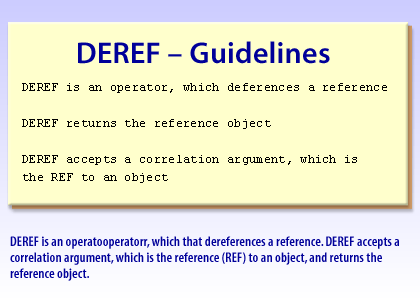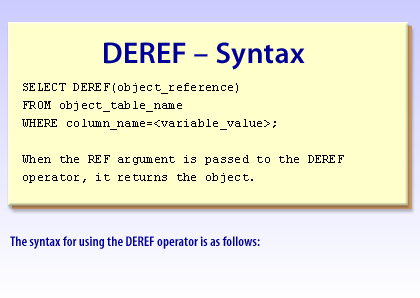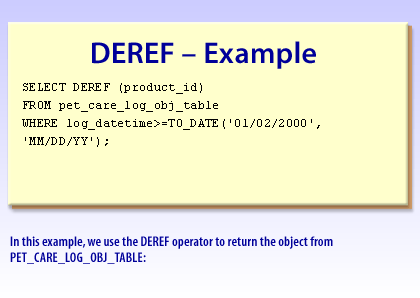PL/SQL   «Prev  Next»
 Lesson 4 Writing queries that use DEREF Objective Write a query using a DEREF data type to retrieve related data.

# Writing Queries that use DEREF in Oracle

In this lesson, we will look at an example that uses a `DEREF` data type to retrieve related data.
`DEREF` is a dereference operator that returns the value of an object. Unlike the `VALUE` operator, it takes a correlation argument, which is a `REF` (reference) to the object. Therefore, if you have a `REF` column within a table and you want to retrieve the target object instead of the reference to it, use `DEREF`. The following series of images shows the syntax for using the `DEREF` data type:1) DEREF is an operator that dereferences a reference. DEREF accepts a correlation argument, which is the reference (REF) to an object, and returns the reference object. DEREF is an operator that dereferences a reference. DEREF returns the reference object. DEREF accepts a correlation argument, which is the reference (REF) to an object2) Syntax for using the DEREF operator is as follows SELECT DEREF(object_reference) FROM object_table_name WHERE column_name=
When the REF argument is passed to the DEREF operator, it returns the object.3) In this example, we use the DEREF operator to return the object from PET_CARE_LOG_OBJ_TABLE: SELECT DEREF (product_id) FROM pet_care_log_obj_tableWHERE log_datetime>=TO_DATE('01/02/2000', 'MM/DD/YY');

## DEREF syntax

DEREF returns the object instance that the REF value points to. This is more easily explained with a simple example. In this case, DEREF is passed the REF value for the correlation ID of the inventory_tbl:

```SELECT DEREF(REF(i))
FROM inventory_tbl i
WHERE item_id = 1;
```

The object instance associated with the REF value of item_id 1 is returned:
```DEREF(REF(I))(ITEM_ID, NUM_IN_STOCK, REORDER_STATUS, PRICE(DISCOUNT_RA
----------------------------------------------------------------------
INVENTORY_OBJ(1, 10, 'IN STOCK', DISCOUNT_PRICE_OBJ(.1, 75))
```

In the next lesson, we will explain when PL/SQL is appropriate or required for querying object tables.
Now that you have learned about the `DEREF` data type, click the link below to learn more about retrieving related data.
Build Deref Query

## Using DEREF in a SELECT INTO Statement

```DECLARE
emp employee_typ;
emp_ref REF employee_typ;
emp_name VARCHAR2(50);
BEGIN
SELECT REF(e) INTO emp_ref FROM employee_tab e
WHERE e.employee_id = 370;
```

The following assignment raises an error, not allowed in PL/SQL
```emp_name := emp_ref.first_name || ' ' || emp_ref.last_name;
emp := DEREF(emp_ref);
```

//is not allowed, cannot use DEREF in procedural statements
SELECT DEREF(emp_ref) INTO emp FROM DUAL; -- use dummy table DUAL emp_name := emp.first_name || ' ' || emp.last_name; DBMS_OUTPUT.PUT_LINE(emp_name); END;
/

Correlation argument: A correlation argument is a parameter, which is passed when a function is used, e.g. the VALUE finction requires the table alias to be passed as a parameter or correlation argument.

PL/SQL Programming